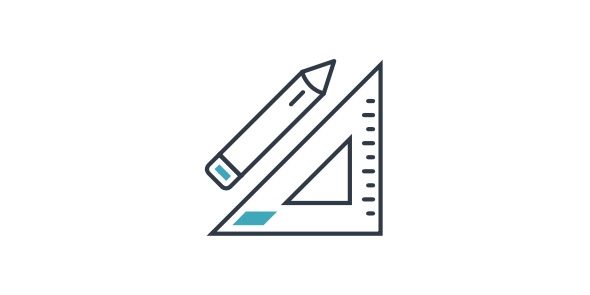# Math Test 1

10 Questions | Total Attempts: 95SettingsThe following quiz is designed to test candidate' s mathematical acumen. The quiz will be offered in a timed mode.

• 1.
What is the value of Pi2?
• A.

22x22/49

• B.

22/70x70

• C.

7x7/22

• D.

70/22x10

• 2.
What does the symbol 'i' mean?
• A.

Infinite

• B.

Indefinite

• C.

Iota

• D.

Inequality

• 3.
If apples cost 50 cents each and oranges cost 20 cents each. What can you buy for 5 dollars and be left with no change?
• A.

8 oranges & 2 apples

• B.

8 apples & 2 oranges

• C.

8 apples & 8 oranges

• D.

8 apples & 5 oranges

• 4.
If x =32, what is the value of 2x-12?
• A.

50

• B.

52

• C.

54

• D.

56

• 5.
If Sam walks 10 miles to north and then another 15 miles to the west, how far is he from the starting point?
• A.

25 miles north west

• B.

5 miles south

• C.

18 miles north east

• D.

18 miles north west

• 6.
If a square is drwan around a circle with radius 4 cms, what will be the area of such a square?
• A.

8 cm sq.

• B.

16 cm sq.

• C.

32 cm sq.

• D.

64 cm sq.

• 7.
If a rectangle is drawn around a circle with radius 4 cms, of which two of the parallel sides are twice the diameter of the circle, and the other two parallel sides are tangential to the circle, what will be the area of such a square?
• A.

128 cm sq.

• B.

160 cm sq.

• C.

320 cm sq.

• D.

640 cm sq.

• 8.
In the series 0,1,3,6,10 what is teh next number?
• A.

12

• B.

15

• C.

18

• D.

21

• 9.
If the population of a city doubles every five years, how much will be the population of the city be in 20 years if the current population is 1000?
• A.

2000

• B.

8000

• C.

16000

• D.

20000

• 10.
For a loan amount of 150,000 how much would be the interst in 20 years at a rate of 5%?
• A.

25000

• B.

87000

• C.

120000

• D.

125000

Related Topics